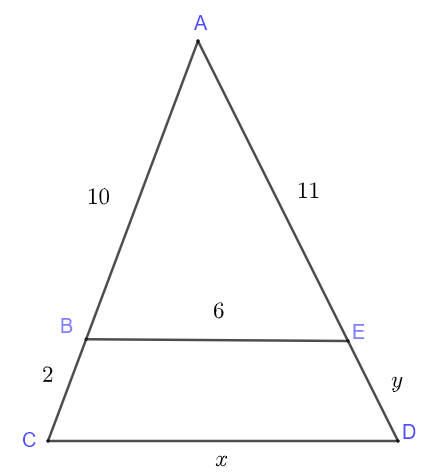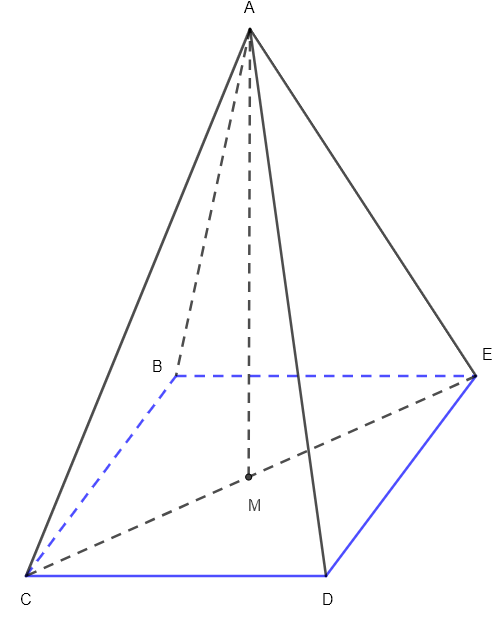# Grade 10 Math Practice Test Questions

Grade 10 math practice test questions including algera, trigonmetry and geometry are presented along with their detailed solutions

1. Question
Solve the systems of equations.2. Question
Expand and simplify the expressions.3. Question
Factor completely the expressions.4. Question

Given $$f(x) = -2 x^2 - 2 x + 4$$, find
a) the vertex of the graph of $$f$$,
b) The x and y intercepts of the graph of function $$f(x) = -2 x^2 - 2 x + 4$$,
c) The axis of symmetry of the graph of $$f$$.
d) Use a calculator to graph $$f$$ and check the answers to parts a) , b) and c).

5. Question
Use proportionality to complete, if possible, the tables of values in a), b) and c).6. Question
Find all unknown sides and angles in the right triangle below.7. Question
Angle $$\alpha$$ is an acute angle such that $$\sin \alpha = 0.6$$. Find $$\cos \alpha$$ and $$\tan \alpha$$

8. Question
In the figure below, BE is parallel to CD. Find the lengths $$x$$ and $$y$$ of the segments CD and DE respectively.9. Question
The lengths of side AB and side BC of a triangle ABC are 14 cm and 10 cm respectively. The size of angle C is 49o. Find all unknown angles and all unknown sides of the triangle.

10. Question
Find the values of $$A$$ and $$B$$ if the line with equation $$A x + By = 1$$ passes through the points $$(1,5)$$ and has a y-intercept at $$y = 3$$.

11. Question
A chemist needs to make 5 L of 45%, by volume, of sulfuric acid solution. He has 20% and 55%, by volume, of sulfuric acid solutions available. He decides to mix the 20% and 55% solutions to make the 45% solution. How many liters of each solution must be mixed?

12. Question
A family drove 1000 km from Paris to Prague in 10 hours. They drove part of the distance at an average speed of 80 km/h, and the rest at an average speed of 120 km/h. What distance did they drive at each speed ?

13. Question
Triangle ABC has vertices at the points $$A(2,3)$$, $$B(-3 , 4)$$ and $$C$$ located on the vertical line $$x = -1$$. Find all possible coordinates of point $$C$$ so that $$ABC$$ is a right triangle with hypotenuse $$AC$$.

14. Question
Linda spends $$70\%$$ of her monthly budget on housing and food and spends $$\ 500$$ more on housing than on food. She spends $$5\%$$ of her budget and that is $$\ 200$$ to pay for a monthly membrship at a yoga club.
How much does she spend on food and housing separately ?

15. Question
Find the area of the kite shown below given that $$\overline {CD} = 10$$ cm16. Question
m and n are parallel lines. Show that lines m and r are perpendicular.17. Question
Find the length of the height AM of the right square pyramid if its volume is 1500 cubic centimeters and the length of the diagonal CE of its base is equal to 10 centimeters.